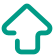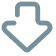# Q - T INTERVAL

QT INTERVAL QT interval : Beginning of Q wave to end of T wave Total duration of Ventricular Systole N/L = 0.35 - 0.44 s ( 1 small square = 0.04 s) Prolonged Q-T interval : Hypokalemia Hypocalcaemia Acute Rheumatic Carditis Acute Myocardial Infarction Hypothermia Congestive Cardiac Failure Cerebro Vascular Accident Quinidine/ Procainamide therapy Shortened Q-T interval : Hypercalcemia Hyperthermia Digitalis therapy Vagal stimulation

(Edited)

1 Like

###### QT interval is standardised at heart rate of 60/min At higher heart rate QT interval decreases, therefore it do not represent the correct QT interval at higher heart rate There are various formulas by which corrected QT interval can be calculated at higher heart rate Corrected QT interval is called as QTc interval The formula are Bazett formula, Fridericia formula, Framingham formula etc###### Agree###### Disagree